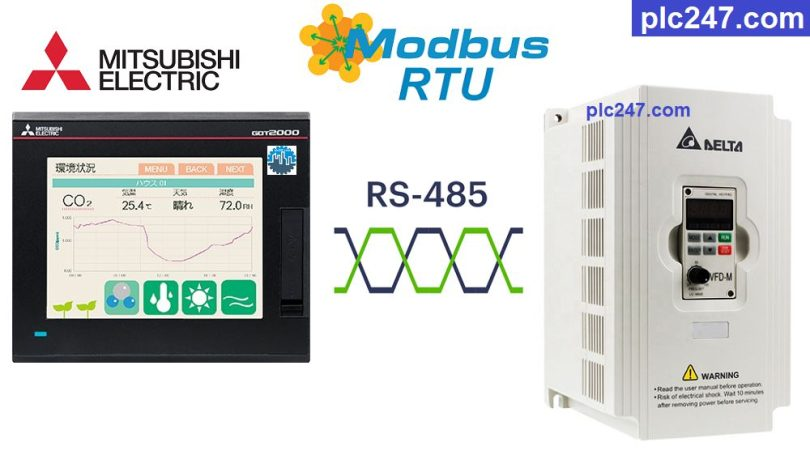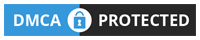# Mitsubishi HMI “Modbus RTU” Delta VFD-M TutorialWritten by

Hello friends!
Delta VFD-M inverter is a type of inverter used extremely commonly in the field of automation, although now this type of inverter has been discontinued and replaced by new types of inverters. However, sometimes we still need to work with them.

So, today plc247.com will guide you to use Mitsubishi GT2505-VTBD HMI to control Delta VFD-M inverter via Modbus RTU (RS485) protocol.

#### HMI Mitsubishi & Delta VFD-M “Wiring Diagram”

+ Use Jack RJ11 (Male) to connect to the RS485 port of Delta VFD-M inverter (which is the jack of the desktop phone)

#### Delta VFD-M Inverter “Modbus” Parameters Setting

In addition to the basic parameters of the inverter installed according to the motor (frequency, voltage, current…) we need to install the following communication parameters:

• P00: 03 (Frequency Set by RS485)
• P01: 04 ( Control by RS485)
• P89: 01 (Baudrate 9600bps)
• P92: 04 (Data =8bit / Stop bit = 1 / Even)
• P157: 01 (Modbus-RTU Selection)
##### Delta VFD-M “Modbus RTU” Registers Address

>>> Control Motor Address = 2000 (hex) = 8192 (dec)+ 400001 = 408193 (dec)

• Set Word 408193 = 0000 0000 0001 0010 (Bin) = 18 (dec) >>> Forward Run
= 0000 0000 0010 0010 (Bin) = 34 (dec) >>> Reverse Run
= 0000.0000.0000.0001 (Bin) = 1 (dec) >>> Stop

>>> Set Frequency Address = 2001 (hex) = 8193 (dec)+ 400001 = 408194 (dec)

• Output Frequency Address = 2103 (hex) = 8451 (dec) + 400001 = 408452 (dec)
• Output Current Address = 2104 (hex) = 8452 (dec) + 400001 = 408453 (dec)
• Output Volatge Address = 2106 (hex) = 8454 (dec) + 400001 = 408455 (dec)

#### HMI GT2505-VTBD Modbus Configuration

How have you installed the communication parameters for the Delta VFD-M inverter, the same settings for the HMI Mitsubishi GT2505

### HMI Modbus Programming

+ Main Control Panel

+ Setting Frequency

+ Motor Forward Run

+ Motor Reverse Run

+ Motor Stop

### Project Video Tutorial

======

References